Chapter 4 Vector Spaces 4 1 Vector spaces

• Slides: 21Chapter 4 Vector Spaces • 4. 1 Vector spaces and Subspaces 向量空间 和 子空间 • 4. 2 Null Space and Column Space 零空间 和 列空间 • 4. 3 Linearly independent sets, bases • 4. 4 Coordinate systems 坐标系 • 4. 5 The dimension of a vector space 维数 • 4. 6 Rank 秩 1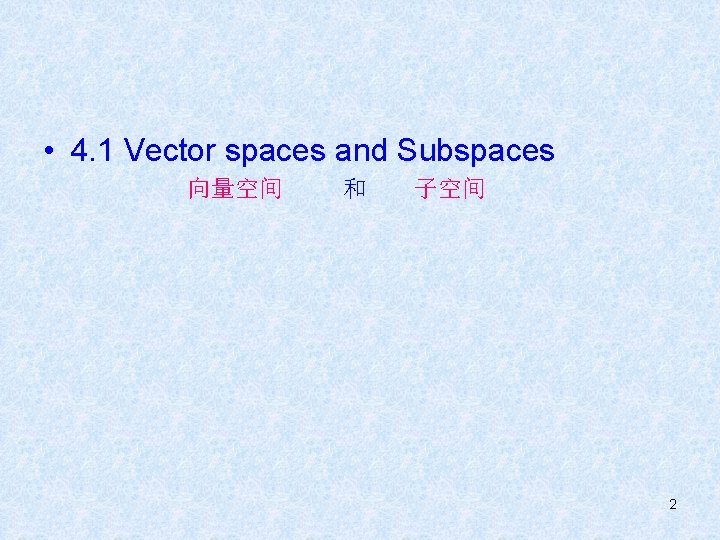• 4. 1 Vector spaces and Subspaces 向量空间 和 子空间 2• DEFINITION • A vector space is a nonempty set V of objects, called vectors, on which are defined two operations, called addition and multiplication by scalars (real numbers), subject to the ten axioms (or rules) listed below. The axioms must hold for all vectors u, v and w in V and for all scalars c and d. 1. The sum of u and v, denoted by u + v, is in V 2. u + v = v + u 3. ( u + v ) + w = u + ( v + w ) 4. There is a zero vector 0 in V, such that u + 0 = u 5. For each vector u in V, there is a vector –u in V, such that u + (–u) = 0 6. The scalar multiple of u by c, denoted by cu, is in V 7. c ( u + v ) = cu + cv 8. ( c + d ) u = cu + du 9. c ( du ) = ( cd ) u 3 10. 1 u = u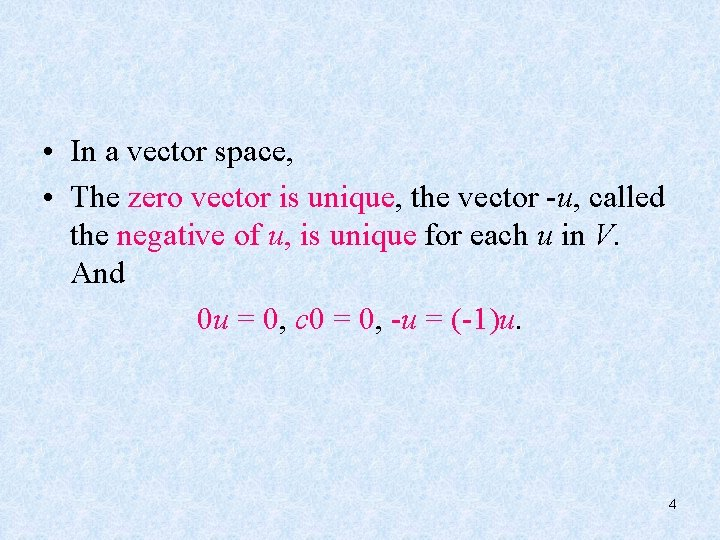• In a vector space, • The zero vector is unique, the vector -u, called the negative of u, is unique for each u in V. And 0 u = 0, c 0 = 0, -u = (-1)u. 456Subspace 子空间 • DEFINITION A subspace of a vector space V is a subset H of V that has three properties: a. The zero vector of V is in H. b. H is closed under vector addition. That is, for each u and v in H, the sum u + v is in H. c. H is closed under multiplication by scalars. That is, for each u is in H and each scalar c, the vector cu is in H. 7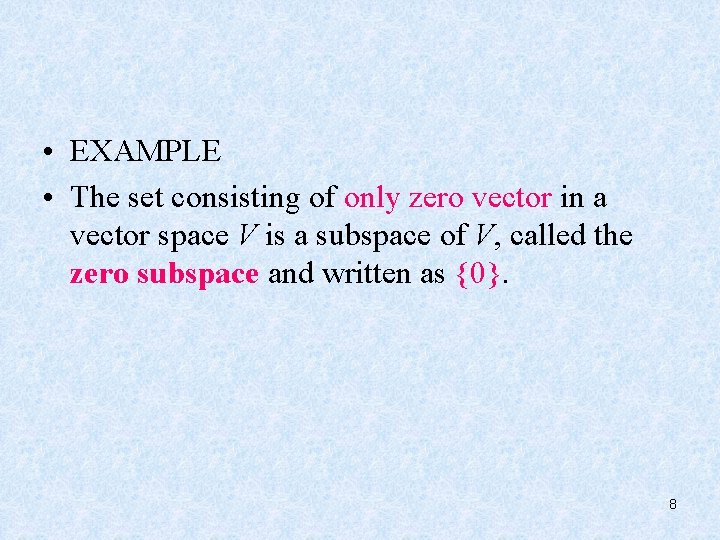• EXAMPLE • The set consisting of only zero vector in a vector space V is a subspace of V, called the zero subspace and written as {0}. 8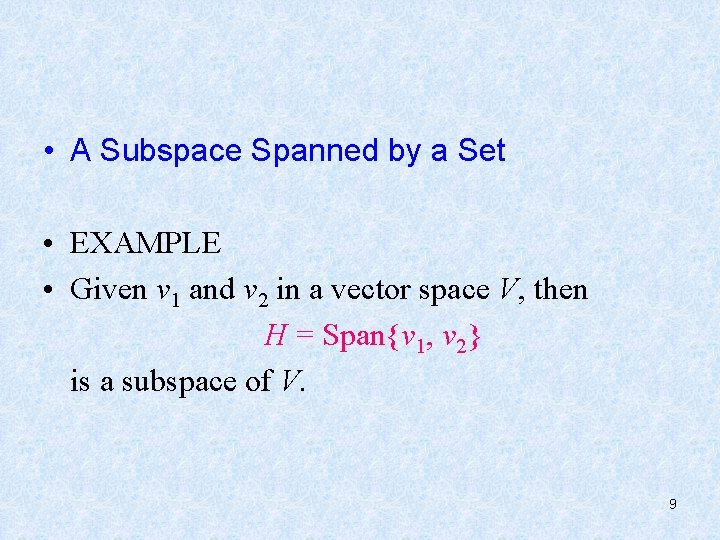• A Subspace Spanned by a Set • EXAMPLE • Given v 1 and v 2 in a vector space V, then H = Span{v 1, v 2} is a subspace of V. 9• THEOREM 1 • If v 1, v 2 , …, vp are in a vector space V, then Span{v 1, v 2 , …, vp } is a subspace of V. 10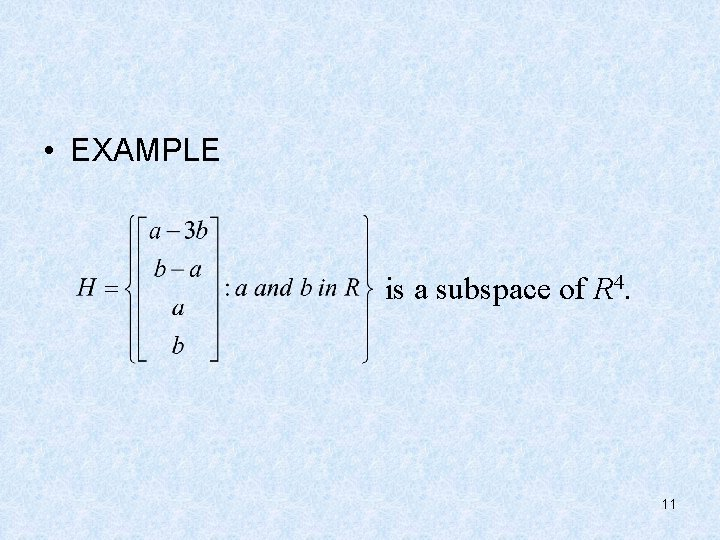• EXAMPLE is a subspace of R 4. 11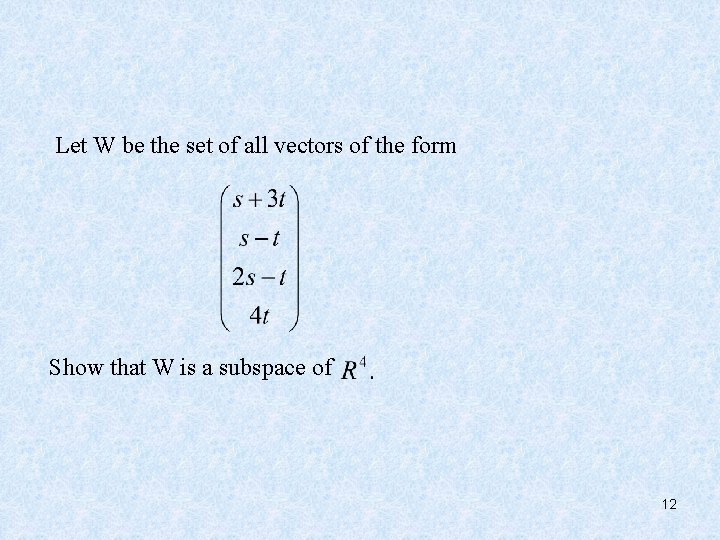Let W be the set of all vectors of the form Show that W is a subspace of 12Let H be the set of all vectors in whose coordinates a, b, c, d satisfy the equations Show that H is a subspace of 13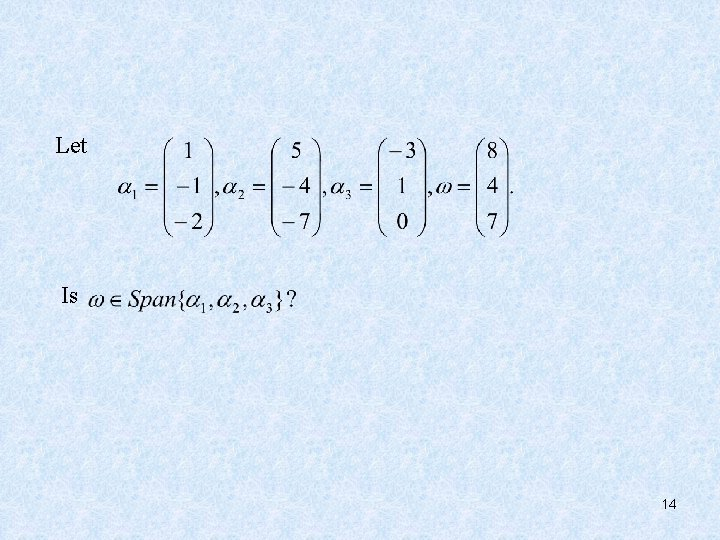Let Is 14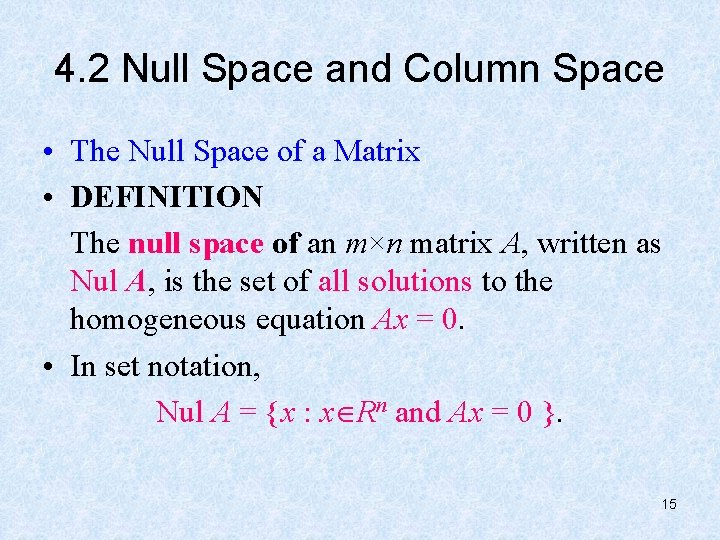4. 2 Null Space and Column Space • The Null Space of a Matrix • DEFINITION The null space of an m×n matrix A, written as Nul A, is the set of all solutions to the homogeneous equation Ax = 0. • In set notation, Nul A = {x : x Rn and Ax = 0 }. 15• THEOREM 2 • The null space of an m×n matrix A is a subspace of Rn. 16So, Nul A = Span{u, v, w} 17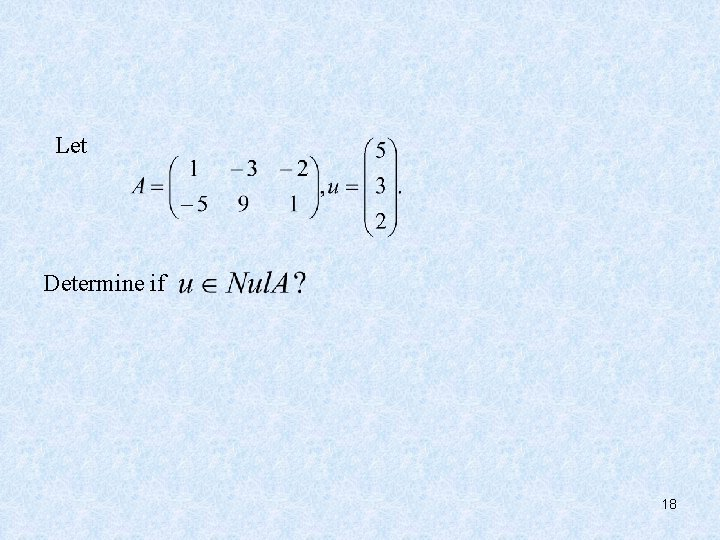Let Determine if 18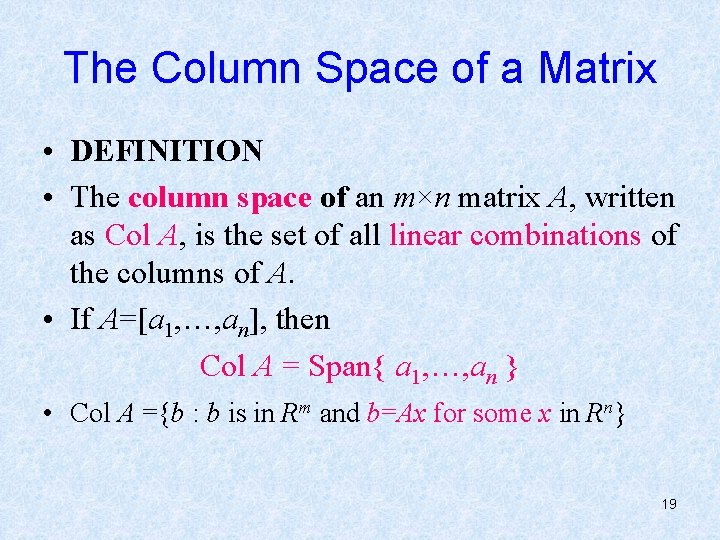The Column Space of a Matrix • DEFINITION • The column space of an m×n matrix A, written as Col A, is the set of all linear combinations of the columns of A. • If A=[a 1, …, an], then Col A = Span{ a 1, …, an } • Col A ={b : b is in Rm and b=Ax for some x in Rn} 19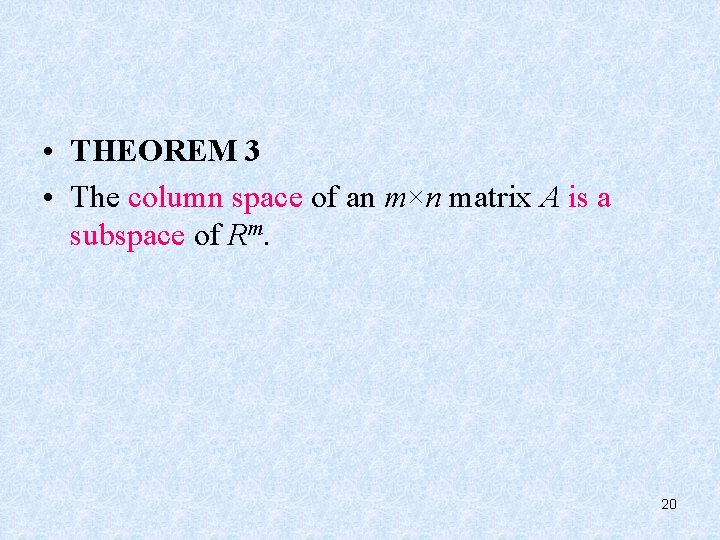• THEOREM 3 • The column space of an m×n matrix A is a subspace of Rm. 20Let Find the spanning set of Nul. A and Col. A. 21